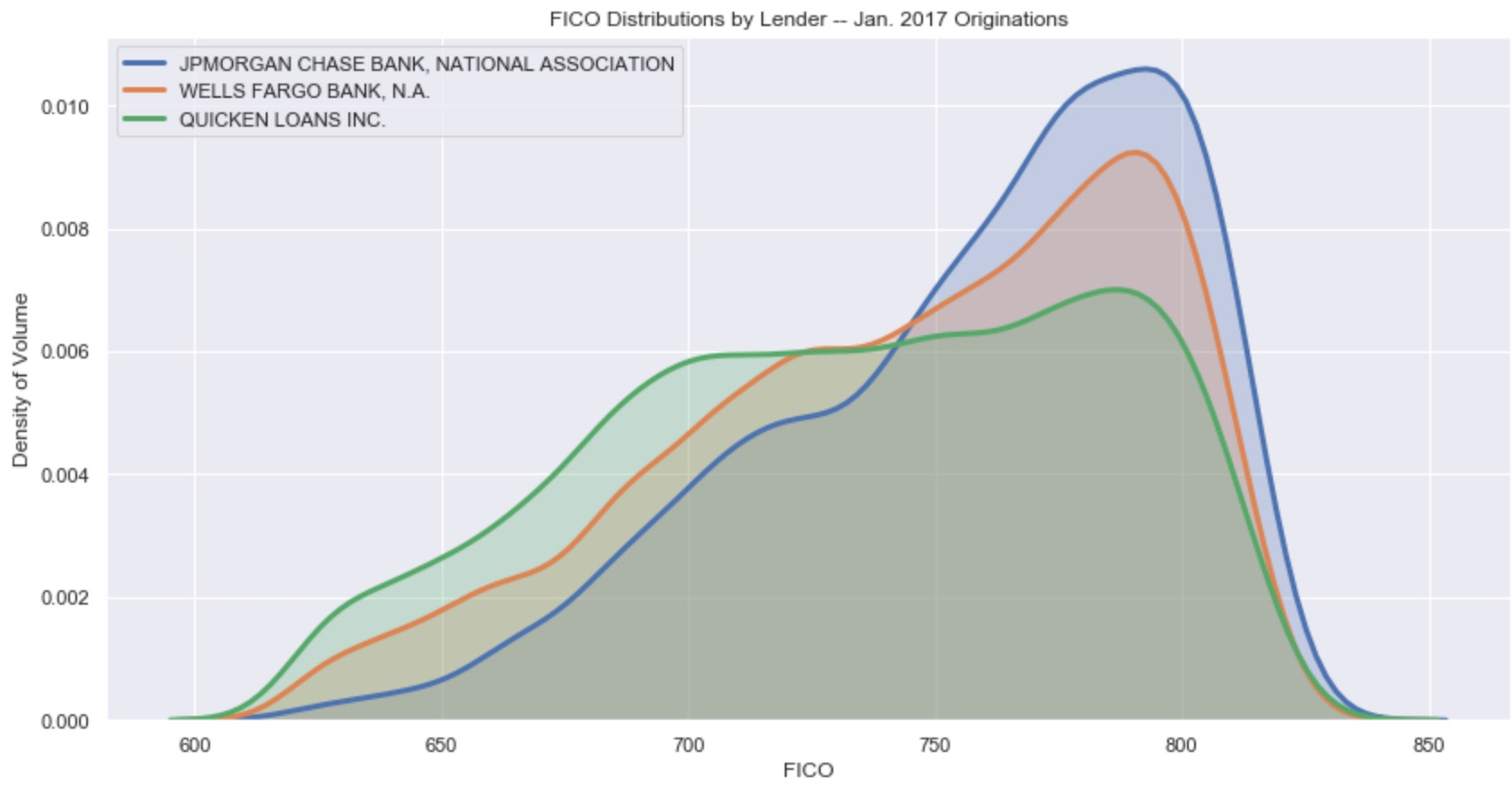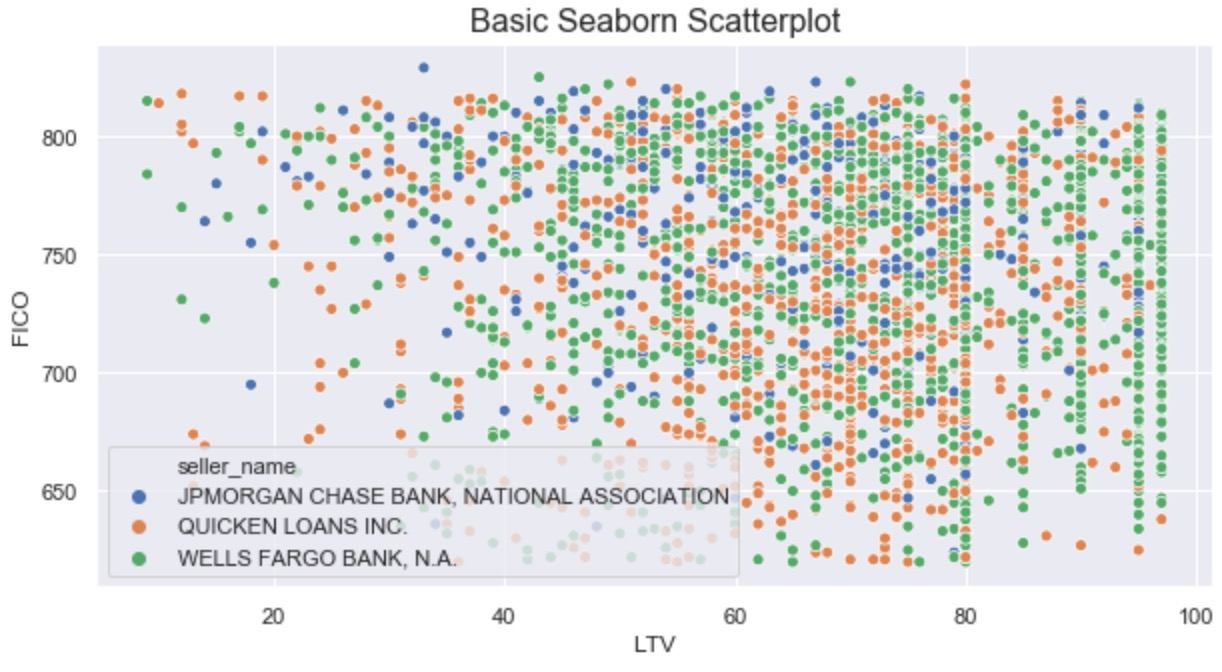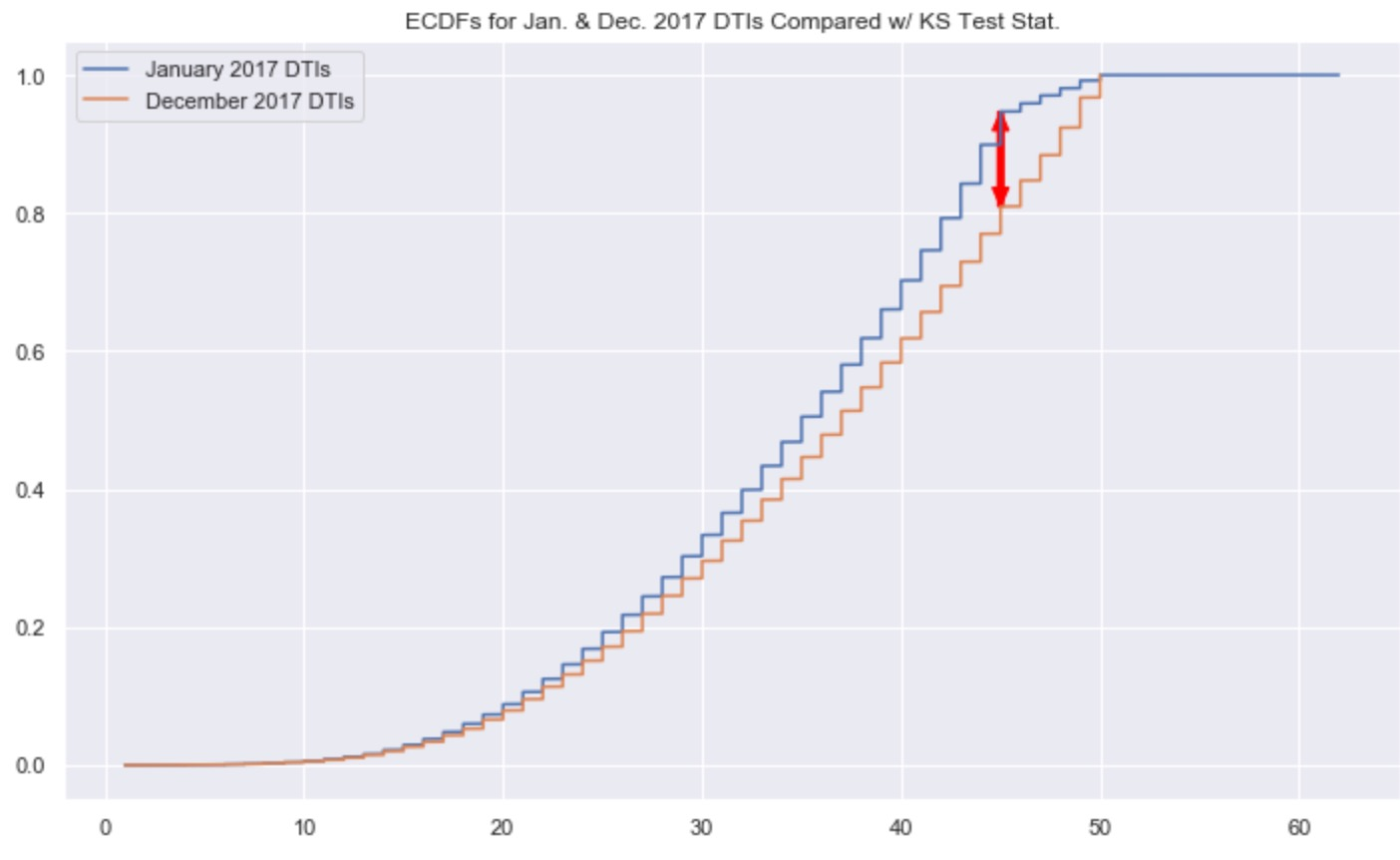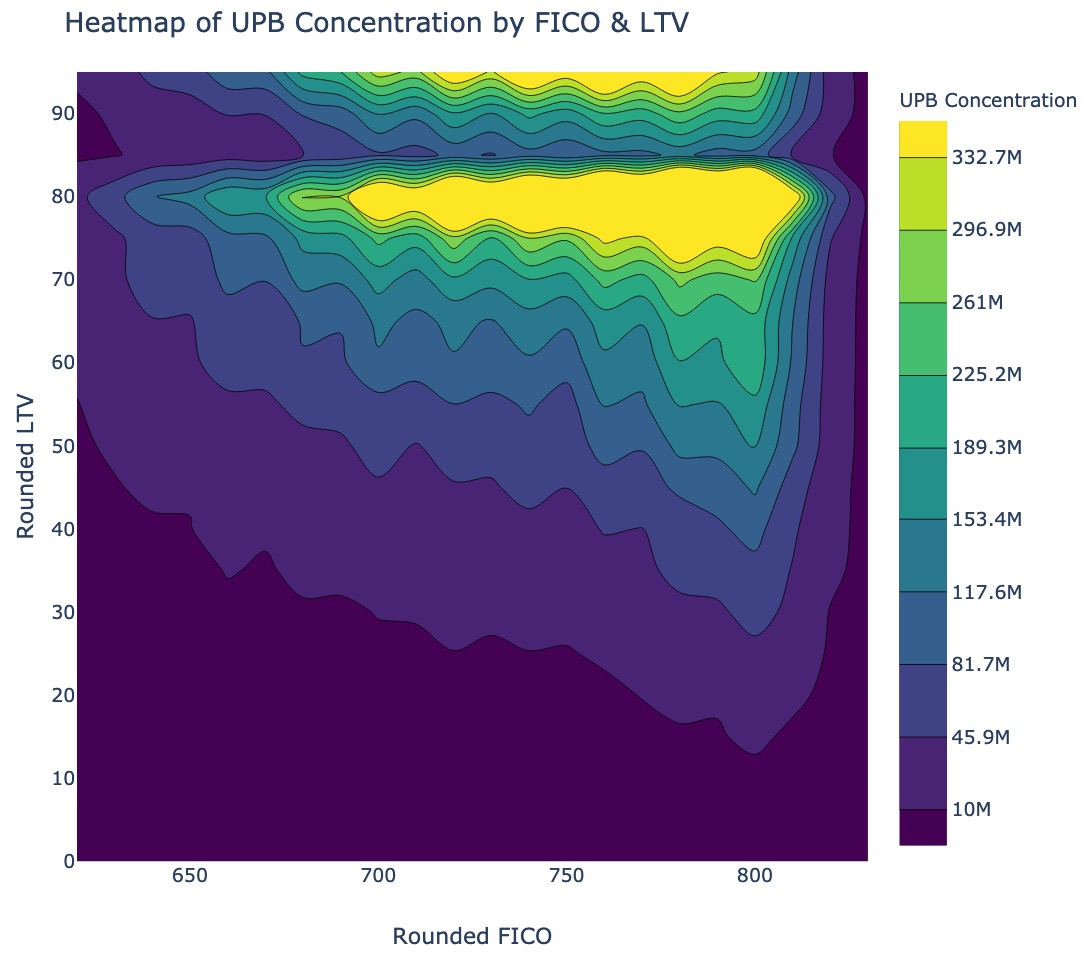# Practical Data Visualization with Python

## Overview

All views expressed on this site are my own and do not represent the opinions of any entity with which I have been, am now, or will be affiliated.

This repository contains all materials related to a lecture / seminar I teach on practical data visualization with python. What I mean by "practical" is that the materials herein do not focus on one particular library or data visualization method; rather, my goal is to empower the consumer of this content with the tools, heuristics, and methods needed to handle a wide variety of data visualization problems.

If you have questions, comments, or suggested alterations to these materials, please open an issue here on GitHub. Also, don't hesitate to reach out via LinkedIn.

## Outline of Materials

Below you'll find a brief outline of the content contained in the four sections of this seminar, along with notebook links, and an example visualization from each section. For each section there is a separate notebook of python code containing all the materials for that section. Each notebook will start with a few setup steps--package imports and data prep mostly--that are almost identical between the notebooks, directly after which comes the content for each section. For information about the data used in these materials, check out the `data_prep_nb.ipynb` notebook, the easy-to-view version of which is hosted here.

### Section 1: Why We Visualize

Here is the link to the easy-to-view notebook for this section of material.
Here is the link to the GitHub-hosted notebook for this section of the material.

1. The power of visual data representation and storytelling.
2. A few principles and heuristics of visualization.
3. The building blocks of visualization explored.

Example Visualization from this Section:### Section 2: Overview of Python Visualization Landscape

Here is the link to the easy-to-view notebook for this section of material.
Here is the link to the GitHub-hosted notebook for this section of the material.

1. Intro to the visualization ecosystem: python's Tower of Babel.
2. Smorgasbord of packages explored through a single example viz.
3. Quick & dirty (and subjective) heuristics for picking a visualization package.

Example Visualization from this Section:### Section 3: Statistical Visualization in the Wild

Here is the link to the easy-to-view notebook for this section of material.
Here is the link to the GitHub-hosted notebook for this section of the material.

1. Example business use case of data visualization:
1. Observational:
• mean, median, and variance
• distributions
2. Inferential:
• parametric tests
• non-parametric tests

Example Visualization from this Section:### Section 4: Library Deep-Dive (Plotly)

Here is the link to the easy-to-view notebook for this section of material.
Here is the link to the GitHub-hosted notebook for this section of the material.

1. Quick and simple data visualizations with Plotly Express.
2. Additional control and complexity with base Plotly.

Example Visualization from this Section:### Homework Exercises

There is a homework associated with these materials, for those interested. Given the open-ended nature of the homework, there is no answer key. That said, if you're working through it and would like some feedback, feel free to reach out to me via LinkedIn.

Here is the link to the easy-to-view homework notebook.
Here is the link to the GitHub-hosted version of the homework notebook.

## Setup Instructions

• clone this repository
• create a virtual environment using `python3 -m venv env`
• activate that virtual environment using `source env/bin/activate`
• install needed packages using `pip install -r requirements.txt`
• run an instance of jupyter lab out of your virutal env using `env/bin/jupyter-lab`
• open and run the four main files of content for this course--one for each section:
• `part_1_main_nb.ipynb`
• `part_2_main_nb.ipynb`
• `part_3_main_nb.ipynb`
• `part_4_main_nb.ipynb`
Alternatives To Practical Python Data Viz Guide
Select To Compare

Alternative Project Comparisons
Related Awesome Lists

Get A Weekly Email With Trending Projects For These Topics
No Spam. Unsubscribe easily at any time.
Python (837,714
Jupyter Notebook (162,988
Material (15,927
Visualization (15,415
Data Science (10,662
Data Visualization (5,915
Data Analysis (5,015
Matplotlib (4,129
Plotting (3,004
Visualisation (2,730
Plotly (1,702
Heatmap (1,587
Bokeh (728
Seaborn (619
Matplotlib Pyplot (231
Altair (87
Plotly Python (41
Plotly Express (28
Matplotlib Tutorial (23
Seaborn Tutorial (13
Plotnine (8